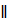# One diagonal of a parallelogram is 70 cm and the perpendicular distance of this diagonal from either of the outlying vertices is 27 cm. The area of the parallelogram (in sq. m) is (a) 1800 (b) 1836 (c) 1890 (d) 1980

## Question ID - 50163 :- One diagonal of a parallelogram is 70 cm and the perpendicular distance of this diagonal from either of the outlying vertices is 27 cm. The area of the parallelogram (in sq. m) is (a) 1800 (b) 1836 (c) 1890 (d) 1980

3537Let ABCD be the givengm.

Let AC = 70 cm.

Draw BLAC, DMAC.

Area ofgm ACBD = ar (ABC) + ar(ACD) == 1890 sq. cm.

Next Question :

Charge is distributed within a sphere of radius R with a volume charge density(r)=e−2r/a  Where A and  are constant. If Q is the total charge of this charge distribution, the radius R is

 a.b.c.d.